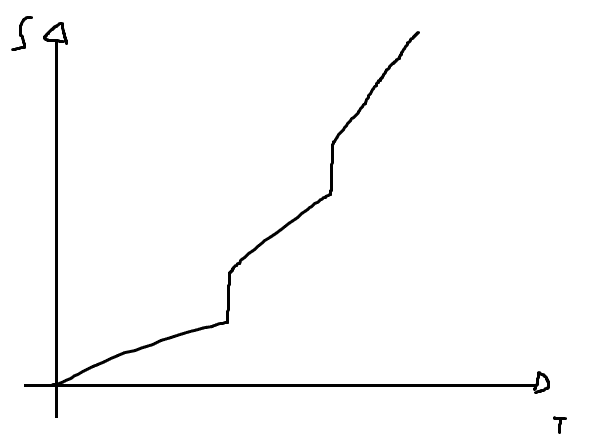# Sketch a qualitatively accurate graph of the entropy of a substance

• LCSphysicist

#### LCSphysicist

Homework Statement
All below
Relevant Equations
All below
Sketch a qualitatively accurate graph of the entropy of a substance
as a function of temperature, at fixed pressure. Indicate where the
substance is solid, liquid, and gas . Explain each feature of the graph briefly.

dU = -P*dV + Q*dS (1)
V = C*T => dV = C*dT
Nfk*dT/2 + P*C*dT = Q*dS

Nfk/2 + P*C = Q*(dS/dT)

For a solid, i assumed f = 3, for a liquid f = 5 and for the gas, f > 5 (i think is not necessary say the obvious this is a rough approximation)That would be the result.

The liquid and gas phases look about right to me (not 100% sure about the solid region). Usually, I see plots of $T$ vs $s$ for liquids and vapours, so I don't want to comment on that (solid) region as I am not too knowledgeable.